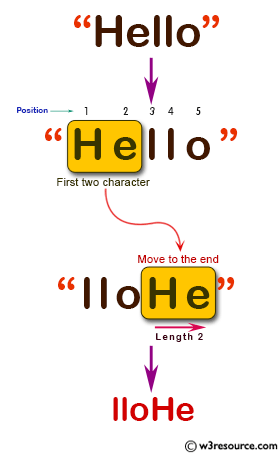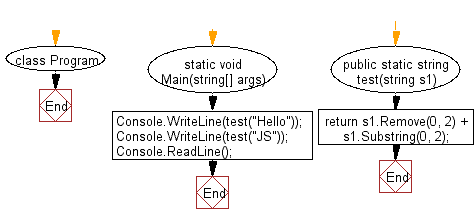﻿ C# Sharp exercises: Move the first two characters to the end of a given string of length at least two - w3resource# C# Sharp Basic Algorithm Exercises: Move the first two characters to the end of a given string of length at least two

## C# Sharp Basic Algorithm: Exercise-68 with Solution

Write a C# Sharp program to move the first two characters to the end of a given string of length at least two.

Pictorial Presentation:Sample Solution:-

C# Sharp Code:

``````using System;

namespace exercises
{
class Program
{
static void Main(string[] args)
{
Console.WriteLine(test("Hello"));
Console.WriteLine(test("JS"));
}

public static string test(string s1)
{
return s1.Remove(0, 2) + s1.Substring(0, 2);
}
}
}
```
```

Sample Output:

```lloHe
JS```

Flowchart:C# Sharp Code Editor:

Improve this sample solution and post your code through Disqus

What is the difficulty level of this exercise?

﻿

New Content: Composer: Dependency manager for PHP, R Programming